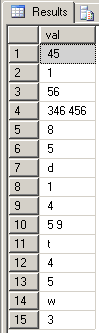SQL Server and T-SQL Development Tutorials Development resources, articles, tutorials, code samples and tools and downloads for ASP.Net, SQL Server, R Script, Windows, Windows Phone, AWS, SAP HANA and ABAP, like SAP UI5, Screen Personas, etc.
 Home Articles News IT Jobs Tools Sample Chapters Trainers Blogs Forums Photos Files

Install SQL Server 2019

# Recursive Function Sample - SQL Server Recursive T-SQL Split Function

Here in this tutorial database developers can find a recursive function sample T-SQL split function which uses recursive CTE (common table expressions) structure in its source code.
If you are working as a SQL Developer or working as an database administrator (DBA), you might probably require a handy t-sql user-defined function, stored procedure or statement that will split an input string (nvarchar data) into pieces according to a given seperator character.
I'm working on my company as a developer and frequently I had to develop t-sql code blocks that will solve problems on SQL Server. T-SQL Recursive Split User-Defined function is one that I programmed for splitting text.

This sample T-SQL recursive split function will work successfully on Microsoft SQL Server 2005 and later versions up to most recent SQL Server 2019.

In addition to given split string SQL functions in this database development tutorial, with SQL Server 2016 SQL developers can use built-in STRING_SPLIT SQL Split String Function

## MSSQL Split Function Example

Here is an example usage of the T-SQL SPLIT function in action

``` declare @s nvarchar(max) select @s = N',,45 , 1, 56, 346 456,8,5, ,d,1,4, 5 9 ,t,,4,5 ,,, w, 3,,' select * from dbo.split(@s,1,0) ```

And you can find the t-sql source code or the sql definition of the T-SQL Recursive Split Function.

``` CREATE FUNCTION SPLIT (   @s nvarchar(max),   @trimPieces bit,   @returnEmptyStrings bit ) returns @t table (id int identity(1,1), val nvarchar(max)) as begin declare @i int, @j int select @i = 0, @j = (len(@s) - len(replace(@s,',',''))) ;with cte as (   select     i = @i + 1,     s = @s,     n = substring(@s, 0, charindex(',', @s)),     m = substring(@s, charindex(',', @s)+1, len(@s) - charindex(',', @s))   union all   select     i = cte.i + 1,     s = cte.m,     n = substring(cte.m, 0, charindex(',', cte.m)),     m = substring(       cte.m,       charindex(',', cte.m) + 1,       len(cte.m)-charindex(',', cte.m)     )   from cte   where i <= @j ) insert into @t (val) select pieces from (   select   case     when @trimPieces = 1     then ltrim(rtrim(case when i <= @j then n else m end))     else case when i <= @j then n else m end   end as pieces   from cte ) t where   (@returnEmptyStrings = 0 and len(pieces) > 0)   or (@returnEmptyStrings = 1) option (maxrecursion 0) return end GO ```I hope this MSSQL split recursive function for splitting strings in sql will be useful for you.

Here is an other version of the above T-SQL split string function which returns a number showing the order of the splitted string.

``` CREATE FUNCTION SPLIT (  @s nvarchar(max),  @splitChar nchar(1) ) returns @t table (id int identity(1,1), val nvarchar(max)) as begin declare @i int, @j int select @i = 0, @j = (len(@s) - len(replace(@s,@splitChar,''))) ;with cte as (  select   i = @i + 1,   s = @s,   n = substring(@s, 0, charindex(@splitChar, @s)),   m = substring(@s, charindex(@splitChar, @s)+1, len(@s) - charindex(@splitChar, @s))  union all  select   i = cte.i + 1,   s = cte.m,   n = substring(cte.m, 0, charindex(@splitChar, cte.m)),   m = substring(    cte.m,    charindex(@splitChar, cte.m) + 1,    len(cte.m)-charindex(@splitChar, cte.m)  )  from cte  where i <= @j ) insert into @t (val) select pieces from (  select  ltrim(rtrim(case when i <= @j then n else m end)) pieces  from cte ) t where  len(pieces) > 0 option (maxrecursion 0) return end GO ```

## SQL String Split Function with Additional Parameters

By adding additional parameters we can empower the SQL string split function to use it in more complex scenarios.
Following user defined function SQLSplitString accepts four parameters:
@string for input concatenated string expression
@seperator for one character seperator to split input string according to
@trim if space characters are not allowed at the beginning and at the end of the splitted pieces
@returnEmptyStrings if the developer wants to eliminate the null or empty string pieces from the returned result set

Here is the source codes of this split string function in SQL

``` CREATE FUNCTION SQLSplitString (  @string nvarchar(max),  @seperator nchar(1),  @trim bit,  @returnEmptyStrings bit ) returns @t table (id int identity(1,1), val nvarchar(max)) as begin declare @i int, @j int select @i = 0, @j = (len(@string) - len(replace(@string,@seperator,''))) ;with cte as (  select   i = @i + 1,   s = @string,   n = substring(@string, 0, charindex(@seperator, @string)),   m = substring(@string, charindex(@seperator, @string)+1, len(@string) - charindex(@seperator, @string))   union all select   i = cte.i + 1,   s = cte.m,   n = substring(cte.m, 0, charindex(@seperator, cte.m)),   m = substring(    cte.m,    charindex(@seperator, cte.m) + 1,    len(cte.m)-charindex(@seperator, cte.m)   )  from cte  where i <= @j ) insert into @t (val) select pieces from (  select  case   when @trim = 1   then ltrim(rtrim(case when i <= @j then n else m end))   else case when i <= @j then n else m end  end as pieces  from cte ) t where  (@returnEmptyStrings = 0 and len(pieces) > 0)  or (@returnEmptyStrings = 1) option (maxrecursion 0) return end GO ```

Related SQL Resources

SQL Server Articles

SQL Server 2012

SQL Server Tools

SQL Blog

MS SQL Server Forums

## SQL Split String Related SQL Server Tutorials

Split String using XML - How to Convert or Split a Delimited String Values to Rows using T-SQL XML Commands
Split String Into Fixed Length Pieces in SQL
Recursive Function Sample - MS SQL Server Recursive T-SQL Split Function
TSQL Character Split Function in SQL Server
Case Sensitive SQL Split Function
SQL Server 2016 Split String Function STRING_SPLIT
SQL Server String Split T-SQL CLR Function Sample
SQL Server CLR Split String Function for 2-Dimensional Array
T-SQL Split User Defined Function